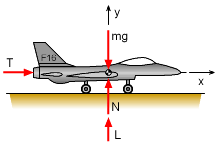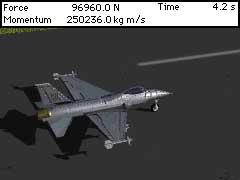Ch 4. Particle Momentum and Impulse Multimedia Engineering Dynamics Impulse & Momentum Consv. Linear Momentum Impact AngularMomentum MassFlow
 Chapter - Particle - 1. General Motion 2. Force & Accel. 3. Energy 4. Momentum - Rigid Body - 5. General Motion 6. Force & Accel. 7. Energy 8. Momentum 9. 3-D Motion 10. Vibrations Appendix Basic Math Units Basic Equations Sections Search eBooks Dynamics Fluids Math Mechanics Statics Thermodynamics Author(s): Kurt Gramoll ©Kurt GramollDYNAMICS - CASE STUDY SOLUTION Solution of a)FBD of F-16 Because the takeoff roll is a straight line, this problem can be analyzed using rectangular coordinates. Begin with a free-body diagram. Let the origin of the rectangular coordinate system be located at the point where the plane begins the takeoff roll, with the positive x axis in the direction of the takeoff roll. Assume there is no force or velocity in the z direction. At t = 0, the velocity of the F-16 is 0,       (vx)t=0 = (vy)t=0 = 0 Use the value of the thrust at t = 0 and t = 5 to express the thrust in the x-direction during the first 5 seconds as a function of time, giving      Tt=0 = 22,250i N        Tt=5 = 111,250i N      T(t) = (22,250 + 17,800t)i N         (0 ≤ t ≤ 5 s) The velocity in the y direction is zero before take off and does not effect the velocity in the x direction. Likewise, forces in the y direction are independent of the thrust before take off. The change in momentum in the x direction is equal to the thrust impulse. This relationship can be used to determine the horizontal velocity for first 5 seconds,The total velocity after the first 5 seconds of the takeoff roll is      vt=5 = 22.86i m/s Solution of b)Take-off Output Data From t = 5 seconds to the time of liftoff (tLO), the thrust is constant and can be expressed as an average constant force,      T = Tavg = 111,250i N        (5 ≤ t ≤ tLO s) For an average or constant force, the impulse integral can be simplified to just force multiple the elapsed time.      (t2 - t1) ΣFx-AV = mvx2 - mvx1      (tLO - 5) (Tavg)x = m (vx)t=LO - m (vx)t=5      (tLO - 5) (111,250) = 14,600 (45.75 - 22.86)      tLO = 8.00 s

Practice Homework and Test problems now available in the 'Eng Dynamics' mobile app
Includes over 400 problems with complete detailed solutions.
Available now at the Google Play Store and Apple App Store.